Next: Input and outputs formats Up: transIT: A Prolog tool Previous: A glimpse of the

# The source and the target frameworks

Let us start by illustrating most of the source languages currently accepted by the translator. The translation of each of them is actually based on specific transformation rules.

Mono-modal logics.
This is the basic translation of modal formulas into relational terms originated in :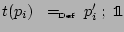,  where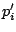is a relational variable uniquely corresponding to, for every propositional variable;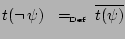,  for every propositional sentence;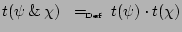,  for all propositional sentences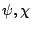;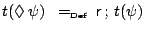,  whereis a constant relation designating the accessibility relation between possible worlds, for every propositional sentence.
Lattice-based modal logics.
These logics, together with their corresponding relational renderings, are described in [14,3,9]. The Prolog translator can handle the modal constructs of
-
lattice-based modal logics with possibility operators;
-
lattice-based modal logics with necessity operators;
-
lattice-based modal logics with sufficiency operators.
Logics of knowledge and information.
These modal logics comes from :
-
Logic with knowledge operator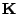, subject to this translation rule: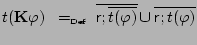-
Logic of non-deterministic information (NIL) [2, Sect. 7.2]. A multi-modal logic with three modalities, representing the relation of inclusion and similarity, proper of an information system.
-
Information logic (IL) [2, Sect. 7.3]. A modal logic with three modal operators corresponding to the relations of indiscernibility, forward inclusion, and similarity of an information system.
Intuitionistic logic.
The translation of intuitionistic logic is based on the following rules: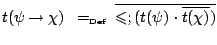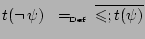whereis a reflexive and transitive relation.
Multi-modal logic.
These logics correspond to multi-modal frames consisting in a relational system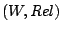where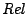is a family of accessibility relations (enjoying closure properties with respect to relational constructs). Modalities are then of the formand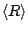where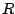is any relational term of.
Other modal logics.
Other modal logics currently accepted by the translator involve: specification operators, temporal operators, Humberstone operators, sufficiency operators, etc .

As concerns the target language to which the translation is directed, currently all translation are performed into the algebra of dyadic relations . So, given a formula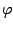the system produces a relational equation of the form=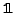.

Further target languages can be easily added by providing suitable description of the languages in terms or rewriting rules. For instance, at the present time, the following targets are under consideration:

• Automated generation of an input file to be processed by deduction systems for relational frameworks. A viable possibility, among others, consists in exploiting a first order theorem prover following the approach described in [6,7];
• Translation into a first order language suitable to be processed by existing deduction systems a la Rasiowa-Sikorski;
• Translation into the language of the theory of aggregates described in [4,1]. In this case, the result of the translation could be inputted to a deduction system for theory-based reasoning ;
• Output in terms of LATEX commands (for both input and output formulas);Next: Input and outputs formats Up: transIT: A Prolog tool Previous: A glimpse of the
Last update: 02-08-2005 by andy